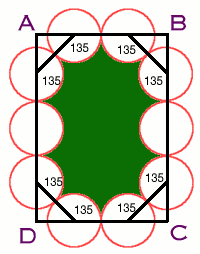#### You may also like### Some(?) of the Parts

A circle touches the lines OA, OB and AB where OA and OB are perpendicular. Show that the diameter of the circle is equal to the perimeter of the triangle### Polycircles

Show that for any triangle it is always possible to construct 3 touching circles with centres at the vertices. Is it possible to construct touching circles centred at the vertices of any polygon?### Circumspection

M is any point on the line AB. Squares of side length AM and MB are constructed and their circumcircles intersect at P (and M). Prove that the lines AD and BE produced pass through P.

# Holly

##### Age 14 to 16 Challenge Level:

James Page of Hethersett High School, Norfolk solved the Holly problem, well done James.

Length $AB = 2 + 2\sqrt2$ cm and length $BC = 4 + 2\sqrt 2$ cm.To find this answer I first took a diagram of the shape and put in the angles. I took 45 from 180 degrees to find the interior angle so I could find the inner curve. I then found that this angle is used 8 times in the diagram. Each circle has a radius of 1 cm and a circumference of $2\pi$ cm. To find the length of the edges of these pieces of the holly I did this:

$\begin{eqnarray} \\ 180 - 45 &=& 135 \\ 135/360 &=& 0.375 \\ (135/360) \times 8 \times (\pi \times 2) &=& 6\pi {\rm cm} \end{eqnarray}$

Then there are the two middle ones of which I had to find the circumference and divide by 2 then multiply by 2 for the two of them, giving a total $2\pi$ cm. I then added the two answers to get the perimeter of the holly leaf which is $8\pi = 25.13$ cm (to 2 decimal places).

If you wished to do a 16 spike holly of the same sort the ends would be the same and it would have an extra 3 circles on each side making the perimeter an extra $6\pi$ cm making a total of $14\pi = 43.98$ cm (to 2 decimal places).

The rectangle $ABCD$ has area

$$(2 + 2\sqrt 2)(4 + 2\sqrt 2) = (16 + 12 \sqrt 2).$$

From this we have to subtract the areas of the four triangles at the corners, a total area of 4 sq. cm., and also the areas of 8 sectors of circles with angles of 135 degrees and the areas of the two semicircles, in all a total area of $4\pi$ sq. cm. The area of the 10 spike holly leaf is

$$16 + 12\sqrt 2 - 4 - 4\pi = 12 + 12\sqrt 2 - 4\pi = 16.40 {\rm cm}^2$$